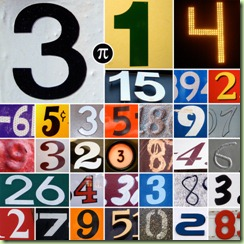## Sunday, March 15, 2009

### Pi day and other ramblingsThe USA congress decided that March the 14th is national Pi day (from chuckchat.com). While I’m not a USA citizen and live nowhere near it, I still thought that the idea is cute. Math can be fun (even though the education systems gives the opposite impression frequently).

A fun fact which I was amazed by when I learned it many years ago: you can calculate Pi probabilistically. The basic idea is to set up an experiment where the probability of the outcome involves Pi. Then perform the experiment a number of times (the higher, the better, since you approximate the actual probability more closely), then go backwards (assume that the actual probability is the one obtained in the experiment and calculate Pi from it). This webpage demonstrates the concept with a Java applet: you put points randomly on a rectangle bounding a circle (technically, one quarter of the circle) and you ask the question: what is the probability of the point ending up inside versus outside of the circle?

The kids on the picture below do a similar thing. Here the question is: what is the probability for the stick frozen hot-dog landing such that it crosses a horizontal line? The math here is slightly more involved, and Pi comes in because you have to take into consideration the angle of the stick hot-dog:

Remaining at the mathematics / fun facts theme, here is a puzzle from abstrusegoose:The site already contains the solution (and so does Google :-)), but it still might be interesting to have a go at it. My solution to the problem (in Perl) can be found in my SVN repo. With this occasion I found out that Math::Prime::XS segfaults, but fortunately the naive algorithms I’ve implemented found the solution quickly.

First picture taken from Mykl Roventine's photostream with permission. The second picture is taken from Jose Kevo's photostream with permission.

Update: changed stick to frozen hot-dog.

1.2.